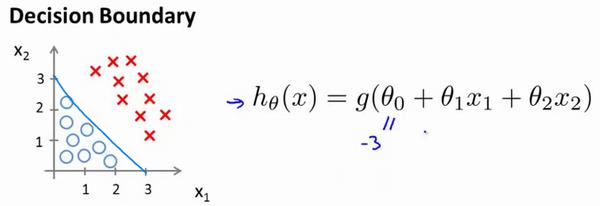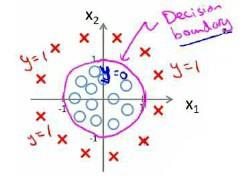# 机器学习1

## 前言

• 数据库挖掘：web数据点击、医疗记录、生物、工程
• 无法手动编程的程序：手写识别、NLP（自然语言处理）、计算机视觉
• 自适应程序：Netflix产品推荐等

• 使计算机自学习
• 从E经验中学习，执行T任务，得出性能衡量P，看是否提高了经验E

## 监督学习（supervised learning）

eg：直线还是二次函数拟合数据模型？给出预测答案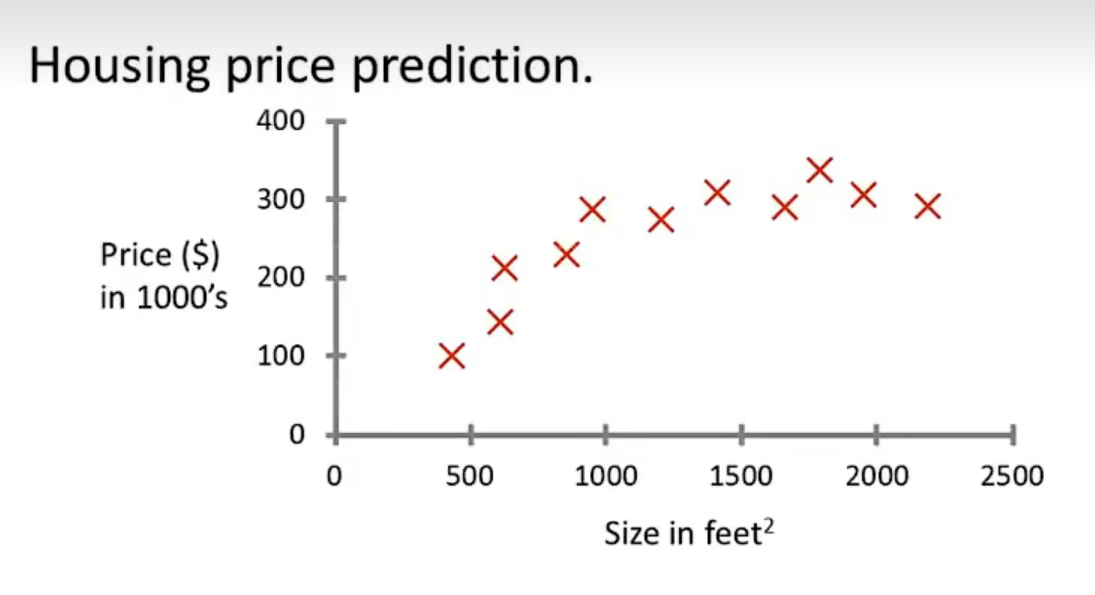## 模型描述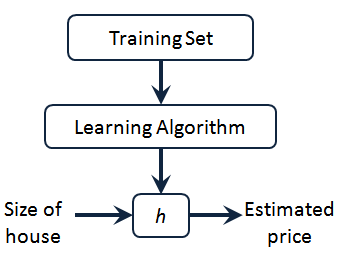h代表假设函数，x$\rightarrow$y

## 代价函数

$h_{\theta}(x) = \theta_{0} + \theta_{1}x$，怎么选择θ0、θ1？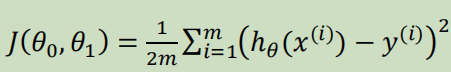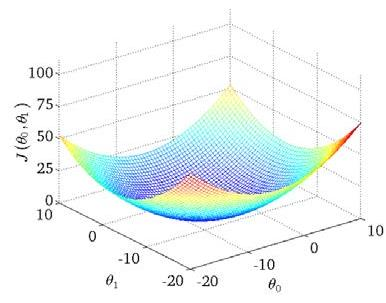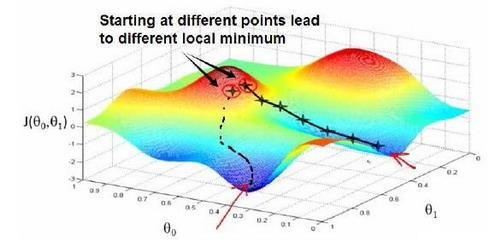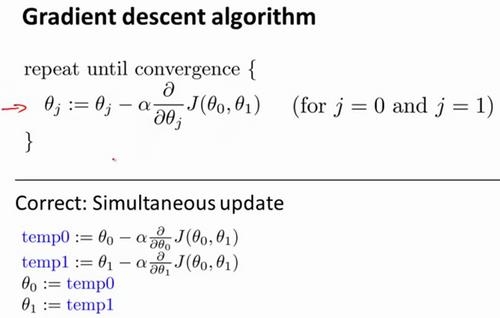α是学习率，更新要在最后做（同步更新）

## 梯度下降的线性回归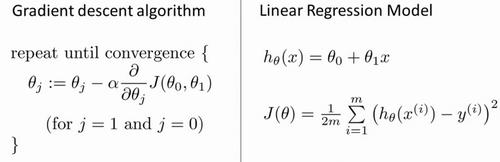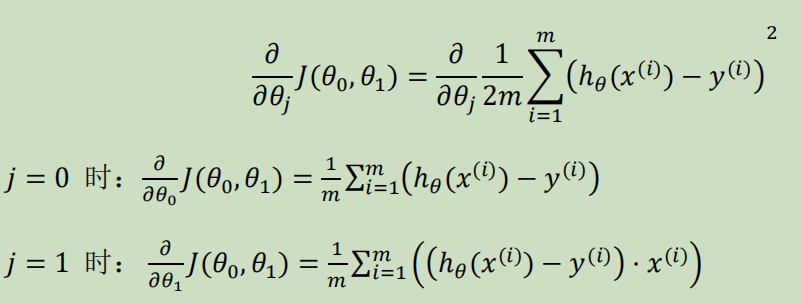## 线性代数回顾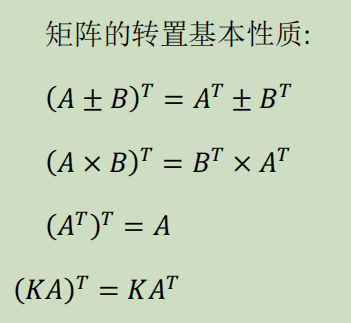## 多变量线性回归

• n代表特征的数量
• $x^{(i)}$代表第i个训练实例（向量）
• $x_{j}^{(i)}$代表第i行第j个特征值

eg：新的假设函数为$h_{\theta}(x)=\theta_0x_0+\theta_1x_2+……+\theta_nx_n$（x0为1），模型的参数为n+1维向量，训练实例也是n+1维向量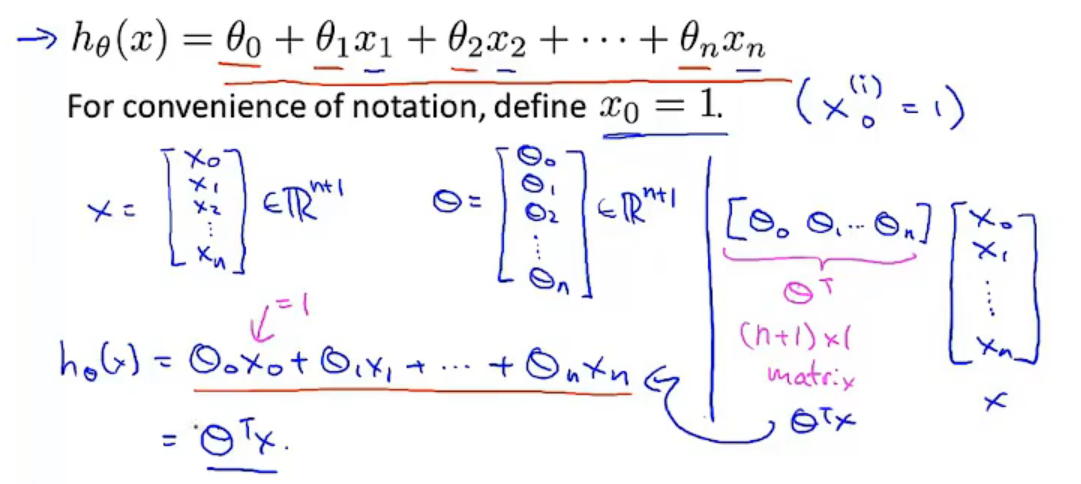### 多元梯度下降法

hθ为上面的假设函数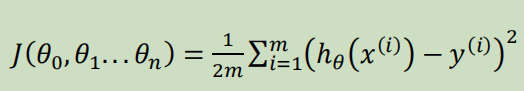### 特征缩放### 特征和多项式回归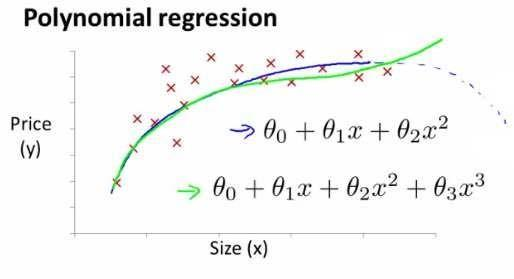### 正规方程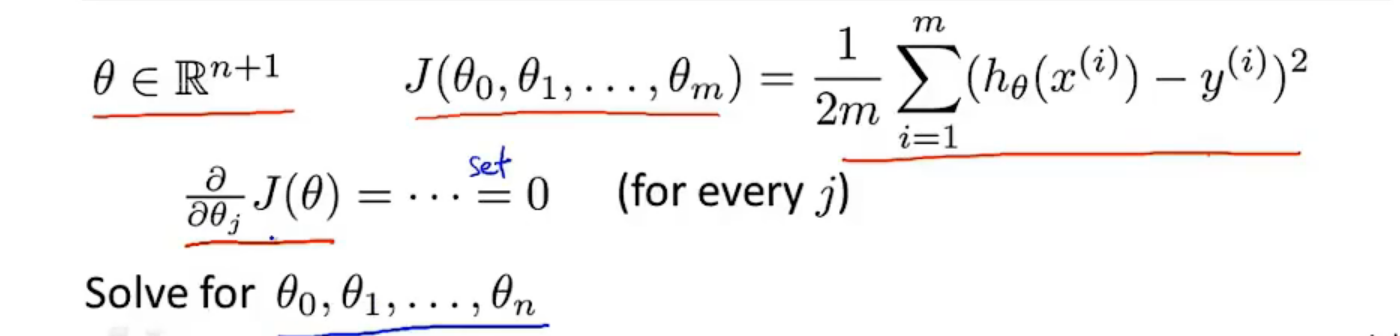### 正规方程 vs 梯度下降

Octave：求解正规方程：pinv(X'*X)*X'*y（伪逆）

$X^TX$为奇异矩阵（不可逆）：删除冗余特征或者正规化特征，解决不可逆问题

## 逻辑回归

### 假设陈述

$h_{\theta}(x)=g(\theta^TX)$代入$g(z)=\frac{1}{1+e^{-z}}$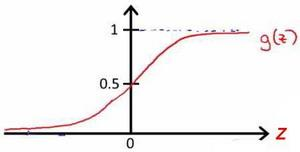### 决策界限